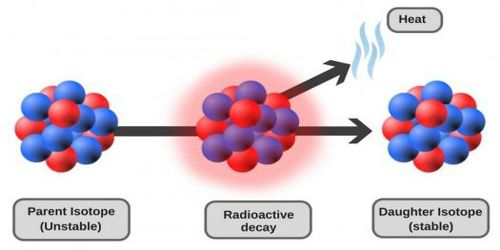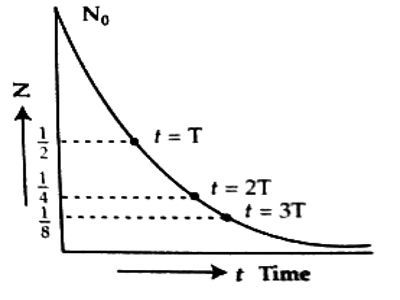Radioactivity is a spontaneous and continuous phenomenon. By the continuous emission of radioactive radiations, a radioactive element is converted into another radioactive element which is also convened or disintegrated into a new element. This process continues unit a new non-radioactive element is produced. During disintegration α or β-rays are emitted. In 1902, Rutherford and Soddy, after extensive studies formulated a law, known as the Law of radioactive disintegration or decay. The law is stated below:

At any moment the number of radioactive atoms that disintegrate in unit time is directly proportional to the number of unchanged radioactive atoms remaining.

If the rate of radioactive disintegration of atoms is dN/dt and if N is the number of unchanged atoms at time t, then – – dN/dt ∞ N or, – dN/dt = constant x N.

or, dN/dt = – λN

or, DN/N = – λ.dt. … … … (1)

Here λ is the radioactive decay constant. Since the number of atoms of radioactive substance decreases, hence a negative sign has been used in the equation.Integrating the equation (1) we get,

loge N = – λt + C … … … (2)

Here C is the constant of integration. Its value is to be determined.

Suppose at the initial time, t = 0, then N = N0.

From equation (2) we get,

loge N0 = C … … … (3)

Now from equations (2) and (3) we get,

logeN = – λt + logeN0.

or, logeN – logeN0 = – λt

or, loge N/N0 = logee-λt

or, N/N0 = e-λt

So, N = N0 e-λt … … …. (4)

This is the law of radioactive decay or disintegration.

This law obeys the exponential law [Figure). Its graph i.e., the graph of time (t) versus unchanged atoms N is shown in the adjoining figure.

From the figure, it is seen that at the start i.e., t = 0, if there is a fixed amount of radioactive atoms then after T its amount decreases by half and after 2T time that residue amount becomes half again. i.e., then it will be ¼ part of the original amount.

From N – t curve it is proved that for total disintegration of a radioactive material it takes infinite time.

Decay constant or disintegration constant:

From equation (4) we get, dN/dt = – λN

or, λ = – (dN/dt) / N.

Now, if N = 1, then from the above equation we get,

λ = – dN/dt.

That means decay constant indicates the probability of decay of an atom in unit time.

Definition: The probability of disintegration or decay of an atom of a radioactive substance in unit time is called the decay constant or disintegration constant.

Its unit is s-1 or day-1 or yr-1.